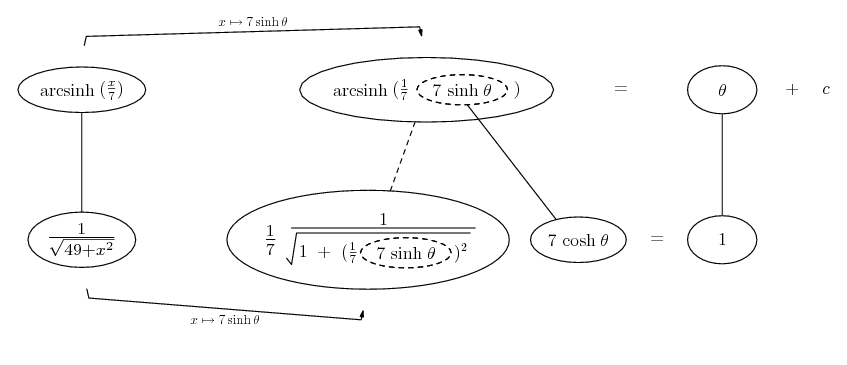# Thread: Trigonometric Substitution Problem

1. ## Trigonometric Substitution Problem

So, I'm trying to solve this problem:and I have x=7tan(theta)
dx=7(sec(theta))^2 d(theta)

Then

INTEGRAL 1/sqrt49+7(tan(theta))^2 * 7(sec(theta))^2 d(theta)

For that integral above, I'm thinking there's an identity to simplify that, but I'm not so sure how to... recognize it?

2. Note the plus sign! Try sinh in place of tan

Edit: pardon the wrong reason - the plus doesn't normally argue against your method, but the integrand is recognisable as the derivative of arcsinh, so we can use sinh.

Just in case a picture is of interest...... where... is the chain rule. Straight continuous lines differentiate downwards (integrate up) with respect to x, and the straight dashed line similarly but with respect to the dashed balloon expression (the inner function of the composite which is subject to the chain rule).

__________________________________________

Don't integrate - balloontegrate!

http://www.ballooncalculus.org/examples/gallery.html

http://www.ballooncalculus.org/asy/doc.html

3.Originally Posted by C.C.So, I'm trying to solve this problem:and I have x=7tan(theta)
dx=7(sec(theta))^2 d(theta)

Then

INTEGRAL 1/sqrt49+7(tan(theta))^2 * 7(sec(theta))^2 d(theta)

For that integral above, I'm thinking there's an identity to simplify that, but I'm not so sure how to... recognize it?
You were on the right track just over looked the simplification in the root and using the identity. Using the substitution tangent.

$\displaystyle \int \frac{1}{\sqrt{49+x^2}}$

$\displaystyle \int \frac{1}{\sqrt{49+(7tan(x))^2}}$

$\displaystyle \int \frac{1}{\sqrt{49+49 tan^2(x)}}$

Now you can use the identity
$\displaystyle \int \frac{1}{\sqrt{49(1+tan^2(x))}}$

Now you can use the identity
$\displaystyle \int \frac{1}{\sqrt{49sec^2(x)}}$

I left out the multiple you use, till I simplified the fraction, to make for a less mess
$\displaystyle \int \frac{7sec^2(x)}{7 sec(x)}{dx}$

You should be able to take it from here, Dont forgot to change your limits of integration like as you had above into terms of theta. hope I didn't lose you on the way and If I made a careless error let me know.4.Originally Posted by C.C.So, I'm trying to solve this problem:and I have x=7tan(theta)
dx=7(sec(theta))^2 d(theta)

Then

INTEGRAL 1/sqrt49+7(tan(theta))^2 * 7(sec(theta))^2 d(theta)

For that integral above, I'm thinking there's an identity to simplify that, but I'm not so sure how to... recognize it?
Just as tom@ballooncalculus noted. One can see that $\displaystyle 49+(7\sinh(x))^2=49\cosh^2(x)$ which is an easier way to do this.

#### Search Tags

problem, substitution, trigonometric